# NEET Previous Year Questions (2014-21): Oscillations Notes | Study Physics Class 11 - NEET

## NEET: NEET Previous Year Questions (2014-21): Oscillations Notes | Study Physics Class 11 - NEET

The document NEET Previous Year Questions (2014-21): Oscillations Notes | Study Physics Class 11 - NEET is a part of the NEET Course Physics Class 11.
All you need of NEET at this link: NEET

Q 1. A body is executing simple harmonic motion with frequency 'n', the frequency of its potential energy is:    
A: 3n
B: 4n
C: n
D: 2n
Ans:
D
Solution:
Displacement equation of SHM of frequency 'n' x = Asin(ωt) = Asin(2πnt) Now,So frequency of potential energy = 2n

Q 2. A spring is stretched by 5 cm by a force 10 N. The time period of the oscillations when a mass of 2 kg is suspended by it is:     
A: 3.14 s
B: 0.628 s
C: 0.0628 s
D: 6.28 s
Ans:

Solution:

F = kx
10 = k(5 × 10–2)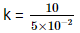= 2 x 102
= 200 N/m
Now,3. Average velocity of a particle executing SHM in one complete vibration is:    
A: Aω/2
B: Aω
C: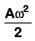D: Zero
Ans:
D
Solution:
In one complete vibration, displacement is zero. So, average velocity in one complete vibration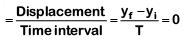Q 4. The displacement of a particle executing simple harmonic motion is given by y = A0+ Asinωt + Bcosωt
Then the amplitude of its oscillation is given by :    
A: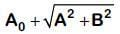B: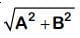C: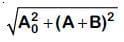D: A + B
Ans:
B
Solution: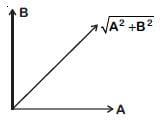y = A0+ Asinωt + Bsinωt
Equate SHM
y' = y – A0 = Asinωt + Bcosωt
Resultant amplitude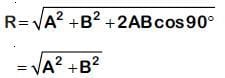Q 5. The radius of circle, the period of revolution, initial position and sense of revolution are indicated in the fig.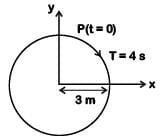y - projection of the radius vector of rotating particle P is:    
A: y(t) = –3 cos2πt, where y in m
B: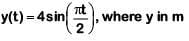C: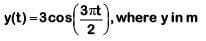D: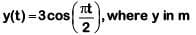Ans:
D
Solution:

At t = 0, y displacement is maximum, so equation will be cosine function.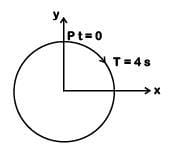T = 4s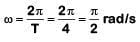y = a cosωt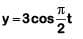Q 6. A pendulum is hung from the roof of a sufficiently high building and is moving freely to and fro like a simple harmonic oscillator. The acceleration of the bob of the pendulum is 20 m/s2 at a distance of 5 m from the mean position. The time period of oscillation is:-    
A: 2πs
B: πs
C: 2s
D: 1s
Ans:
B
Solution: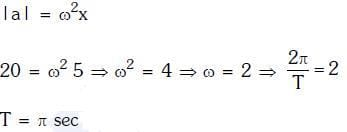Q 7. A particle executes linear simple harmonic motion with an amplitude of 3 cm. When the particle is at 2 cm from the mean position, the magnitude of its velocity is equal to that of its acceleration. Then its time period in seconds is:-    
A: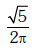B: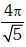C: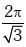D: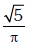Ans:
B
Solution: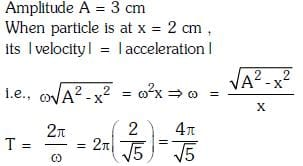Q 8. A particle is executing SHM along a straight line. Its velocities at distances x1 and x2 from the mean position are V1 and V2, respectively. Its time period is:    
A: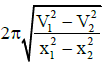B: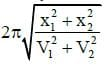C: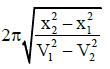D: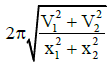Ans: C
Solution: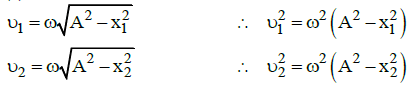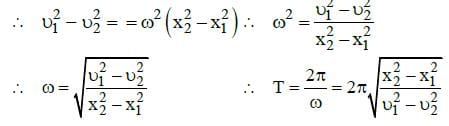Q 9. When two displacements represented by y1 = a sin(ωt) and y2 = b cos(ωt) are superimposed the motion is:    
A: simple harmonic with amplitude (a+b)/2
B: not a simple harmonic
C: simple harmonic with amplitude a/b
D: simple harmonic with amplitude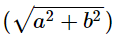Ans:
D
Solution: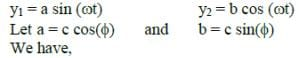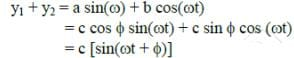where  c2 = a2 + b2  [since  a+ b2 = c2 cos (φ) + csin (φ) = c2 ]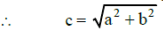The superimposed motion is simple harmonic with amplitude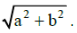Q 10. The oscillation of a body on a smooth horizontal surface is represented by the equation,
X = A cos (ωt)
Where X = displacement at time t
ω = frequency of oscillation
Which one of the following graphs shows correctly the variation ‘a’ with ‘t’?    
A: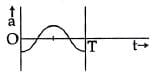B: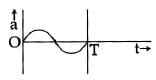C: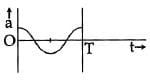D: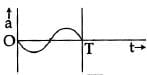Here, a = acceleration at time ‘t’ and T = time period
Ans:
A
Solution: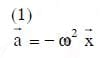The document NEET Previous Year Questions (2014-21): Oscillations Notes | Study Physics Class 11 - NEET is a part of the NEET Course Physics Class 11.
All you need of NEET at this link: NEETUse Code STAYHOME200 and get INR 200 additional OFF

## Physics Class 11

80 videos|393 docs|210 tests

### How to Prepare for NEET

Read our guide to prepare for NEET which is created by Toppers & the best Teachers

Track your progress, build streaks, highlight & save important lessons and more!

,

,

,

,

,

,

,

,

,

,

,

,

,

,

,

,

,

,

,

,

,

;